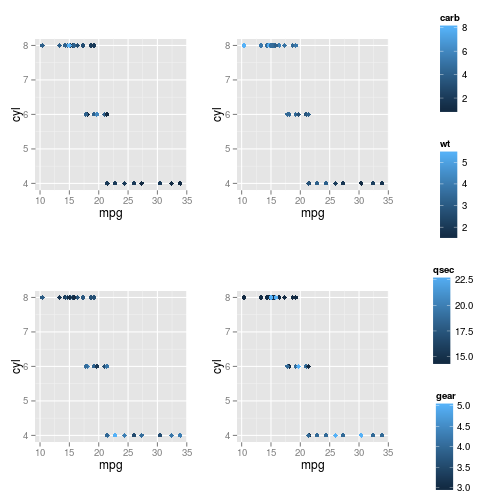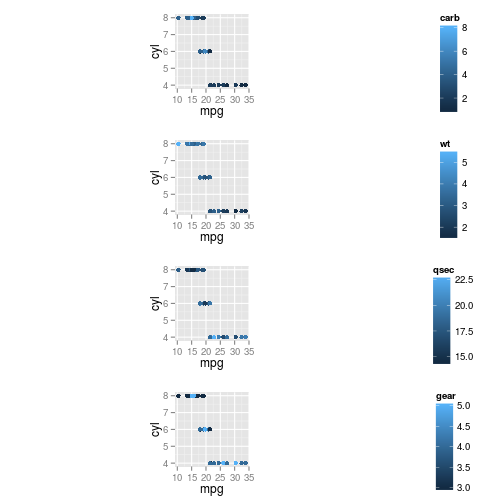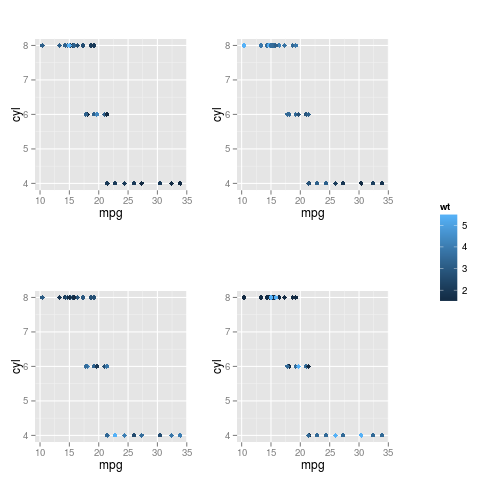arrangeGrobByParsingLegend {ggbio} R Documentation

## Arrange grobs by parse their legend.

### Description

Arrange grobs and parse their legend, then put it together on the right.

### Usage

```arrangeGrobByParsingLegend(..., nrow = NULL, ncol = NULL,
widths = c(4, 1), legend.idx = NULL)
```

### Arguments

 `...` ggplot graphics. `nrow` number of row for layout. `ncol` number of columns for layout `widths` width ratio for plot group and legend group. `legend.idx` legend index you want to keep.

a

Tengfei Yin

### Examples

``````library(ggplot2)
p1 <- qplot(x = mpg, y= cyl, data = mtcars, color = carb)
p2 <- qplot(x = mpg, y= cyl, data = mtcars, color = wt)
p3 <- qplot(x = mpg, y= cyl, data = mtcars, color = qsec)
p4 <- qplot(x = mpg, y= cyl, data = mtcars, color = gear)
arrangeGrobByParsingLegend(p1, p2, p3, p4)
``````
``````## NULL
````````````arrangeGrobByParsingLegend(p1, p2, p3, p4, ncol = 1)
``````
``````## NULL
````````````arrangeGrobByParsingLegend(p1, p2, p3, p4, legend.idx = 2)
``````
``````## NULL
``````[Package ggbio version 1.5.20 ]